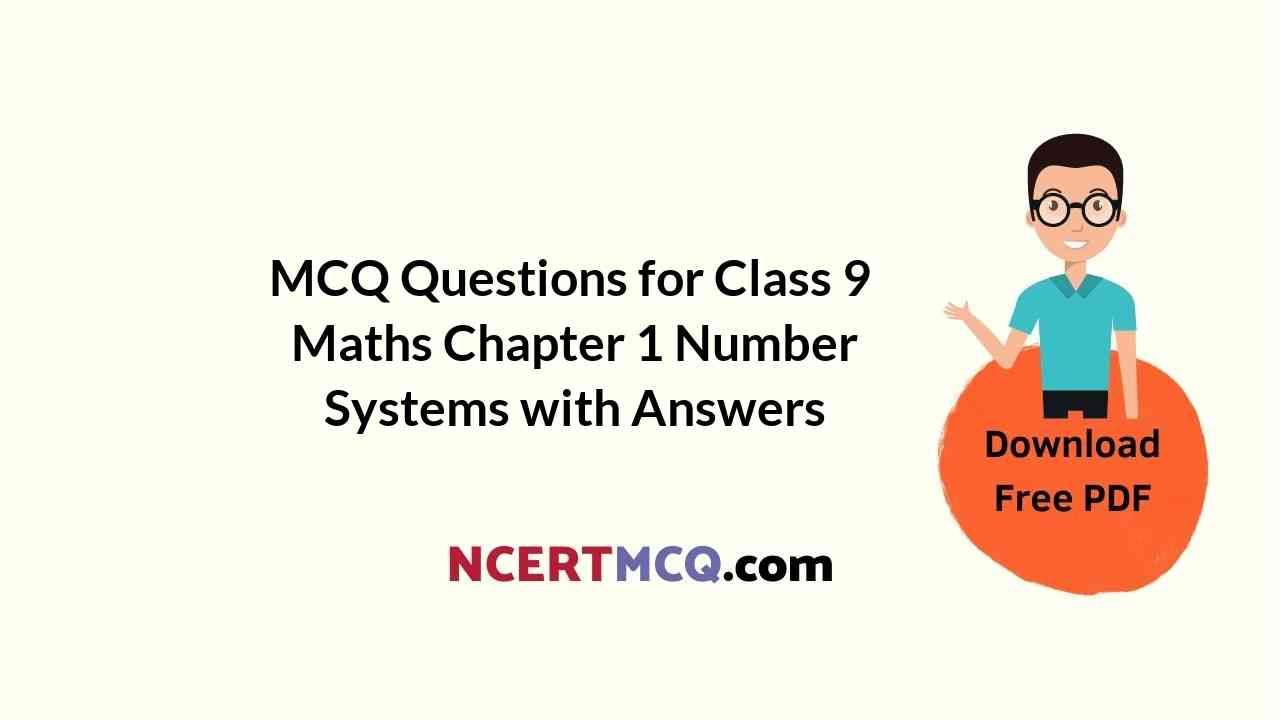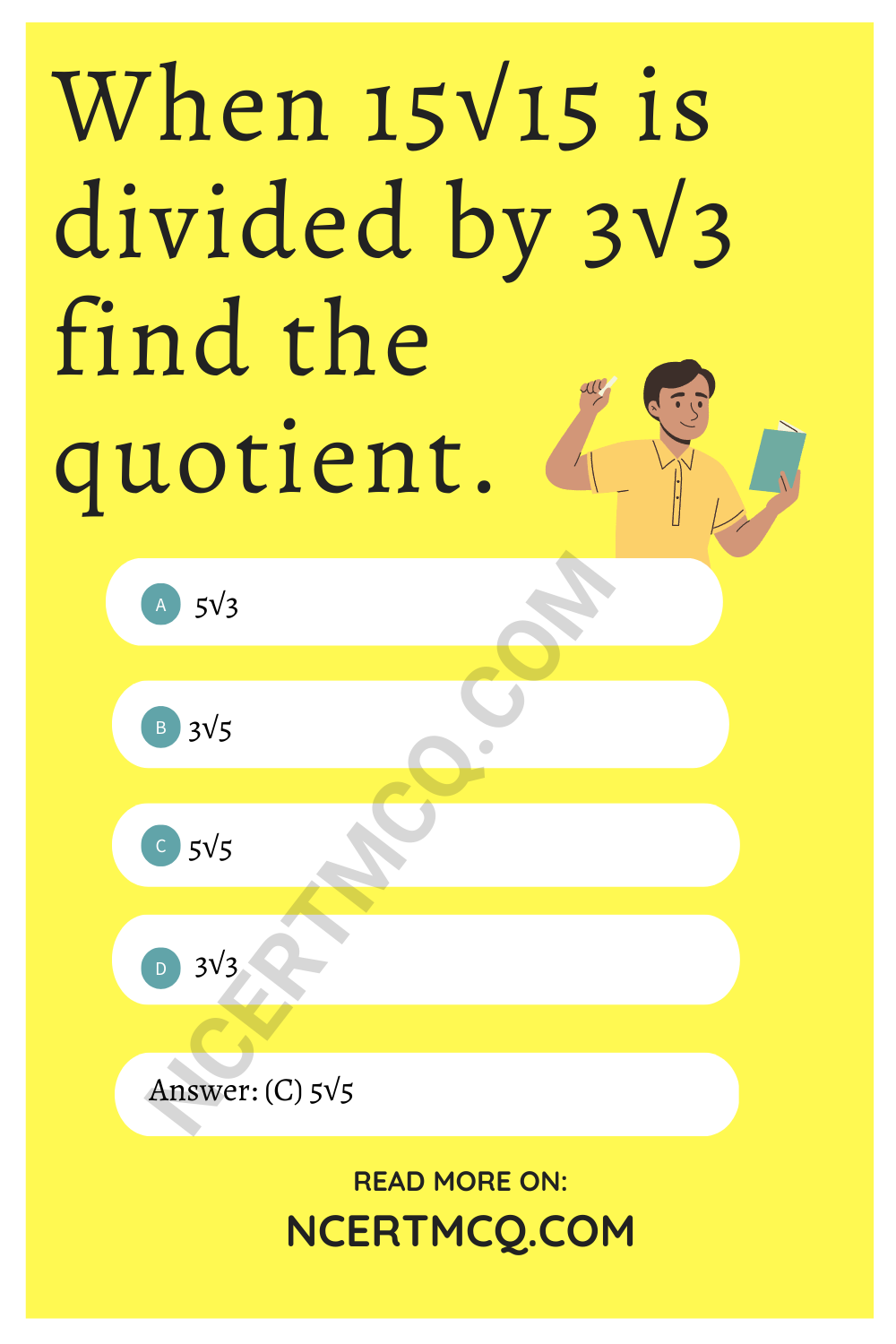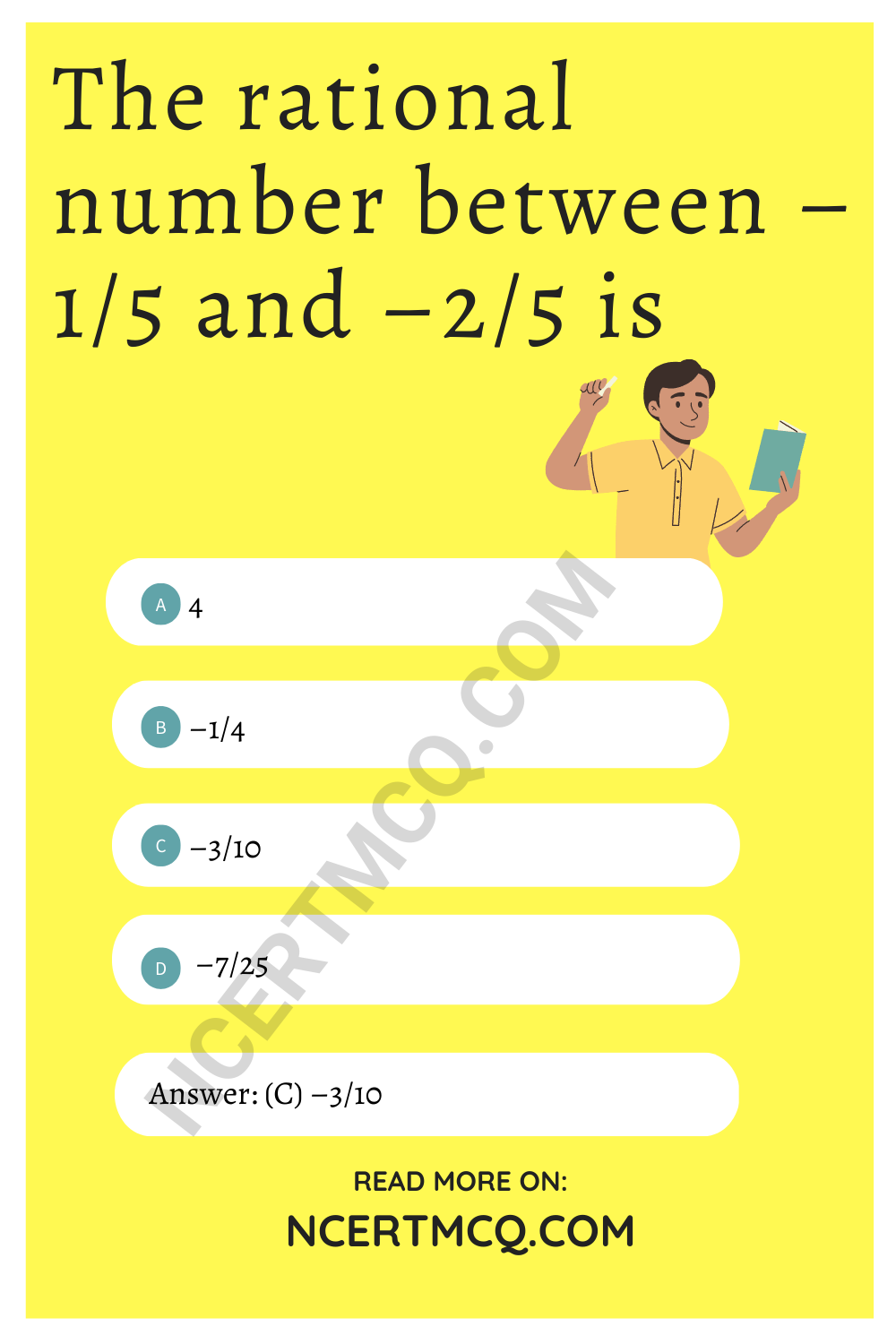Check the below Online Education NCERT MCQ Questions for Class 9 Maths Chapter 1 Number Systems with Answers Pdf free download. MCQ Questions for Class 9 Maths with Answers were prepared based on the latest exam pattern. We have provided Number Systems Class 10 Maths MCQs Questions with Answers to help students understand the concept very well. https://ncertmcq.com/mcq-questions-for-class-9-maths-with-answers/

Students can also refer NCERT Solutions for Class 9 Maths Chapter 1 Number System for better exam preparation and score more marks.

## Number Systems Class 9 MCQs Questions with Answers

Number System Class 9 MCQ Question 1.
The value of $$\sqrt{\sqrt{2^{2}}}$$ is equal to
(a) 2–$$\frac{1}{6}$$
(b) 2-6
(c) 2$$\frac{1}{6}$$
(d) 26

Answer: (c) 2$$\frac{1}{6}$$

Class 9 Maths Chapter 1 MCQ Question 2.
Find the value of $$\sqrt{\frac{54}{250}}$$
(a) $$\frac{9}{25}$$
(b) $$\frac{3}{5}$$
(c) $$\frac{27}{125}$$
(d) $$\frac{\sqrt2}{5}$$

Answer: (b) $$\frac{3}{5}$$

Number System MCQ Class 9 Question 3.
Simplified value of (16)–$$\frac{1}{4}$$ × $$\sqrt{16}$$ is
(a) 16
(b) 4
(c) 1
(d) 0

Class 9 Number System MCQ Question 4.
Find the value of $$\sqrt{64^{-2}}$$.
(a) $$\frac{1}{8}$$
(b) $$\frac{1}{2}$$
(c) 8
(d) $$\frac{1}{64}$$

Answer: (a) $$\frac{1}{8}$$

Class 9 Maths Ch 1 MCQ Question 5.
Find the value of $$\sqrt{216}$$ – $$\sqrt{125}$$
(a) 1
(b) -1
(c) $$\sqrt{91}$$
(d) $$\frac{6}{5}$$

Class 9 Maths Chapter 1 MCQ With Answers Question 6.
When 15$$\sqrt{15}$$ is divided by 3√3 find the quotient.
(a) 5√3
(b) 3√5
(c) 5√5
(d) 3√3Ch 1 Maths Class 9 MCQ Question 7.
If √3 = 1.732 and √2 = 1.414, find the value of $$\frac{1}{√3-√2}$$
(a) 0.318
(b) 3.146
(c) $$\frac{1}{3.146}$$
(d) $$\sqrt{1.732}$$ – $$\sqrt{1.414}$$

Maths Class 9 Chapter 1 MCQ Question 8.
Which of the following numbers is an irrational number?
(a) $$\sqrt{16}$$ – 4
(b) (3 – √3) (3 + √3)
(c) √5 + 3
(d) –$$\sqrt{25}$$

MCQ Of Number System Class 9 Question 9.
The decimal expansion of √2 is
(а) finite decimal
(b) 1.4121
(c) non-terminating recurring
(d) non-terminating non-recurring

MCQ On Number System Class 9 Question 10.
If x = $$\frac{√7}{5}$$ and $$\frac{5}{x}$$ = p√7, then the value of p is
(а) $$\frac{5}{√7}$$
(b) $$\frac{25}{7}$$
(c) $$\frac{7}{25}$$
(d) $$\frac{√7}{5}$$

Answer: (b) $$\frac{25}{7}$$

Chapter 1 Maths Class 9 MCQ Question 11.
The sum of 0.$$\bar{3}$$ and 0.$$\bar{2}$$ is
(а) $$\frac{5}{99}$$
(b) $$\frac{5}{9}$$
(c) $$\frac{5}{10}$$
(d) $$\frac{5}{100}$$

Answer: (b) $$\frac{5}{9}$$

MCQ Class 9 Maths Chapter 1 Question 12.
(√a + √b) (√a – √b) is
(a) a + b
(b) a – b
(c) 2√a
(d) 2√b

Class 9 Math Chapter 1 MCQ Question 13.
$$\sqrt{12}$$ × √8 is equal to
(a) 2√6
(b) 3√6
(c) 4√6
(d) 6√6

Class 9 Chapter 1 Maths MCQ Question 14.
Find the value of $$\frac{2^0+7^0}{5^0}$$
(a) 2
(b) 0
(c) $$\frac{9}{5}$$
(d) $$\frac{1}{5}$$

MCQ Questions For Class 9 Maths Chapter 1 Question 15.
A rational number lying between √2 and √3 is
(a) $$\frac{√2+√3}{2}$$
(b) √6
(c) 1.6
(d) 1.9

Class 9th Maths Chapter 1 MCQ Question 16.
The rational number between –$$\frac{1}{5}$$ and –$$\frac{2}{5}$$ is
(a) 4
(b) –$$\frac{1}{4}$$
(c) –$$\frac{3}{10}$$
(d) –$$\frac{7}{25}$$

Answer: (c) –$$\frac{3}{10}$$Class 9 Maths MCQ Chapter 1 Question 17.
If 8x = $$\frac{64}{2^x}$$ then find the value of x.
(a) 4
(b) 2
(c) $$\frac{1}{2}$$
(d) $$\frac{3}{2}$$

Answer: (d) $$\frac{3}{2}$$

MCQ Of Maths Class 9 Chapter 1 Question 18.
The simplified value of (81)–$$\frac{1}{4}$$ × $$\sqrt{81}$$ × (81)0.2 is
(a) 9
(b) 3
(c) 1
(d) 0

Maths Chapter 1 Class 9 MCQ Question 19.
Value of [(81-1/2)-1/4]² is
(a) 3
(b) $$\frac{1}{3}$$
(c) 9
(d) $$\frac{1}{9}$$

MCQ Questions For Class 9 Maths Number System With Answers Question 20.
If x1/12 = 491/24 then find the value of x.
(a) 49
(b) 2
(c) 12
(d) 7